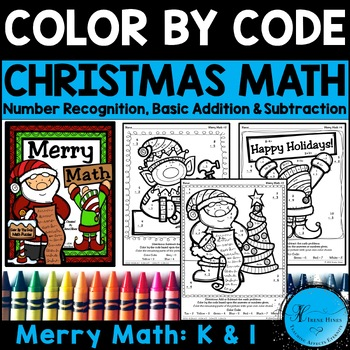DID YOU KNOW:
Seamlessly assign resources as digital activities

Learn how in 5 minutes with a tutorial resource. Try it Now

Learn More# Christmas Merry Math Activities: Addition & Subtraction Color By The Number CodeK - 1st, Homeschool
Resource Type
Formats Included
• PDF
Pages
11 pages

### Description

Christmas Math Activities ~ Merry Math ~ Color By The Number Code Puzzle Printables For December, Christmas and Winter To Practice Number Recognition Skills And Basic Addition And Subtraction Facts

~This Color By Number Unit Is Aligned To The CCSS. Each Page Has The Specific CCSS Listed.~

This set includes 4 Christmas themed math puzzles to practice math skills. Perfect for Kindergarten and First Grade Math.

Skills Covered:

~ Number Recognition
~ Basic Subtraction Facts

Details Of CCSS Skills Covered:

CCSS.Math.Content.K.OA.A.1 Represent addition and subtraction with objects, fingers, mental images, drawings, sounds (e.g., claps), acting out situations, verbal explanations, expressions, or equations.

CCSS.Math.Content.K.OA.A.5 Fluently add and subtract within 5.

CCSS.Math.Content.1.OA.C.5 Relate counting to addition and subtraction (e.g., by counting on 2 to add 2).

CCSS.Math.Content.1.OA.C.6 Add and subtract within 20, demonstrating fluency for addition and subtraction within 10. Use strategies such as counting on; making ten (e.g., 8 + 6 = 8 + 2 + 4 = 10 + 4 = 14); decomposing a number leading to a ten (e.g., 13 – 4 = 13 – 3 – 1 = 10 – 1 = 9); using the relationship between addition and subtraction (e.g., knowing that 8 + 4 = 12, one knows 12 – 8 = 4); and creating equivalent but easier or known sums (e.g., adding 6 + 7 by creating the known equivalent 6 + 6 + 1 = 12 + 1 = 13).

Set also includes 4 answer keys for the 4 puzzles.

Click on the preview to see the puzzles.

~~~~~~~~~~~~~~~~~~~~~~~~~~~~~~~~~~~~~~~~~~~~~~~~~~~~~~~~~

~Here Are Some More December Units To Check Out!~

Monthly Math Masterpieces~ Winter Edition Pack Of Math Puzzles

Bright Ideas For The Holidays ~ Christmas Math Color By The Code

December Division De'lights' ~ Math Color By The Code Puzzles

Christmas Computation Cupcakes ~ Addition And Subtraction Math Color By The Code Puzzles

Multiplication Christmas Computation Cupcakes ~ Math Color By The Code Puzzles

Christmas Elf Equations : Christmas Addition Math Printables

Multiplication Snowflake Solutions ~ Math Winter Printables Color By The Code Puzzles

Merry Math: Addition & Subtraction Color By The Number Code Christmas Puzzles To Practice Number Recognition Skills And Basic Addition And Subtraction Facts

Language "Art" : Winter Edition ~ Seasonal Color By The Code Printable Puzzles For Language Arts

The Biggest Christmas Tree Ever Book Unit

The Biggest Snowman Ever Book Unit

Christmas Combo Pack: Two Units In One…Language Arts and Mathematics!

December And Winter Writing Prompt Calendar

Daily Journal Writing Prompts ~ December And Winter

~~~~~~~~~~~~~~~~~~~~~~~~~~~~~~~~~~~~~~~~~~~~~~~~~~~~~~~~~

Are you interested in getting ALL of the “Color-By-The-Code” Math Puzzles in my store?

By popular demand, I have put every one of my math puzzles together for one purchase! This bundle option also gives you lifetime access of every new math puzzle I create with nothing more to buy ever!!

Math Puzzle Palooza: My Entire Store Of "Color-By-The-Code" Math Puzzles!

** If you like color by the code puzzles, but you do not want to buy the bundle, you might be interested in my individual packets which are listed here:

My Complete Color By The Code Math Puzzle List

~~~~~~~~~~~~~~~~~~~~~~~~~~~~~~~~~~~~~~~~~~~~~~~~~~~~~~~~~

~ If you would like to be notified when my products are updated, freebies are offered and new items are added to my store, then follow me! ~

Thank you!

Irene Hines

Teaching Affects Eternity

Total Pages
11 pages
Included
Teaching Duration
1 Week
Report this Resource to TpT
Reported resources will be reviewed by our team. Report this resource to let us know if this resource violates TpT’s content guidelines.